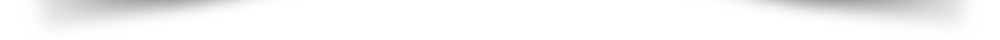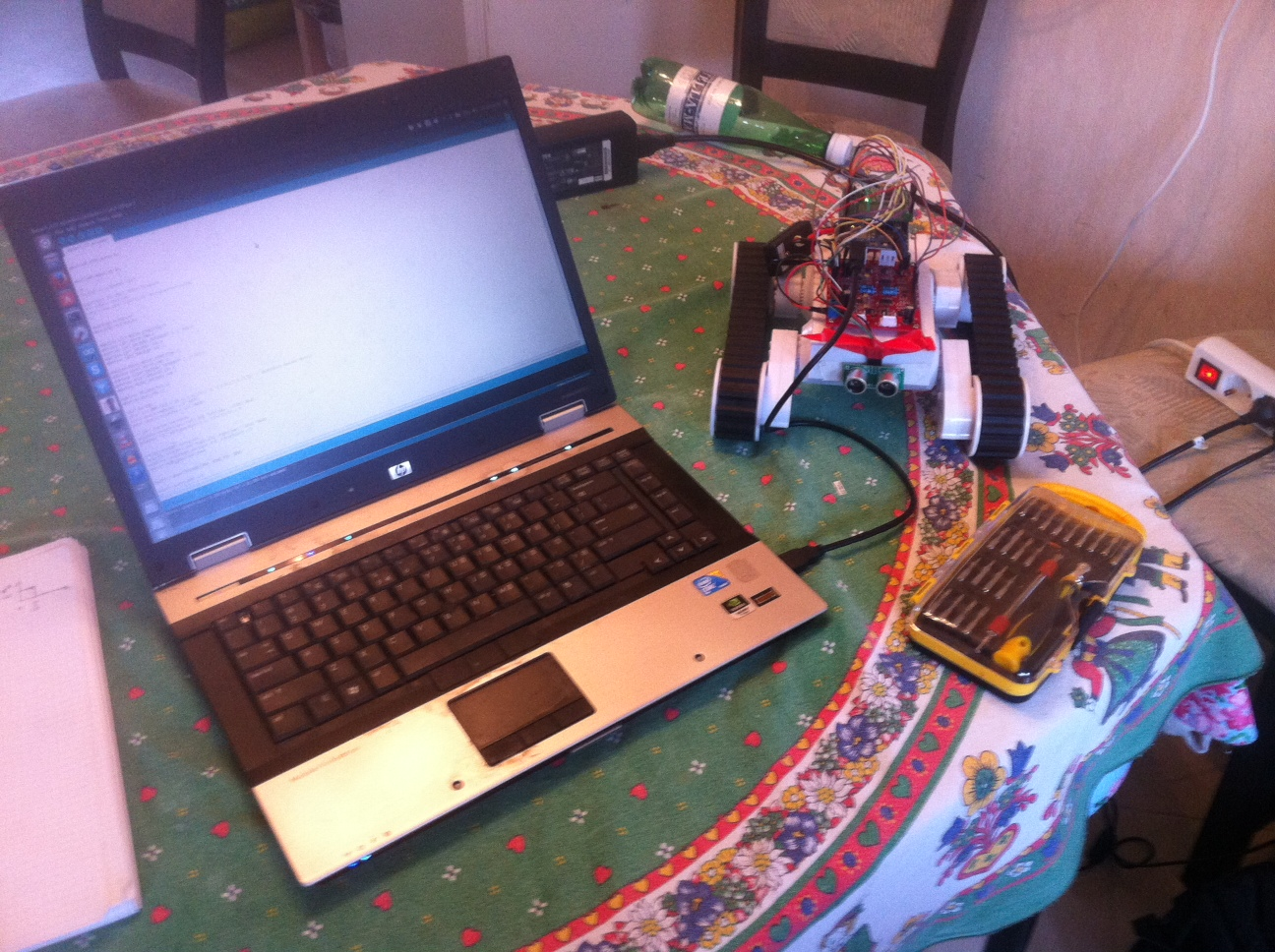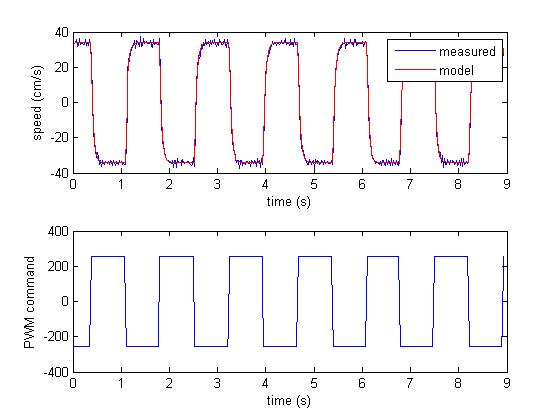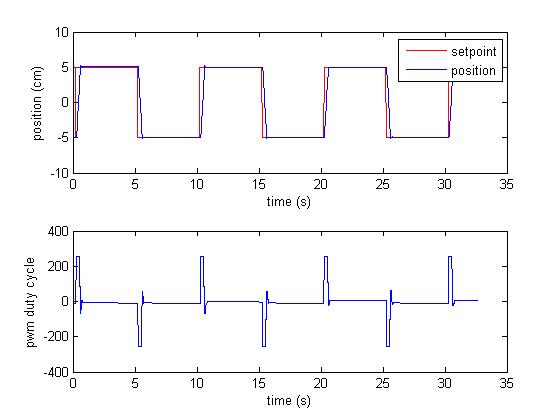## Rover 5 + Arduino position control project

The Rover 5 is an easy-to-use robot chassis designed for students and hobbyist. The Arduino UNO is a popular single-board microcontroller based on a 8-bit Atmel AVR microcontroller. In this project, I make use of the Arduino microcontroller and some basic concepts of System Identification and Control in order to command a Rover 5 robot chassis .The Rover 5 robot chassis, my laptop, and a set of screwdrivers laying on my kitchen table.

### Objective

An ultrasonic distance sensor is used to measure the distance between the robot and the closes object around. The robot will try to keep this distance constant. If I put your hand close to the sensor, the robot will move back and forward giving the impression of following the hand. A movie that shows the final result can be found here .

### Materials

The following materials are required for this project:
• A Rover 5 "ROV5-2" robot chassis (2 motors, 2 encoders)
• An Arduino UNO REV 3 microcontroller
• A Dagu V12 4 Channel Motor Control Unit for Rover 5
• 6 AA NiMh 1.2V batteries
• Ultrasonic distance sensor US-020 (or similar)
• A Bluetooth communication shield BTV2.1 for arduino (optional).
• Male to male and male to female jumper wires, PVC plastic, a power switch, screws, etc.
Most of the components above were purchased online from www.dx.com. Tools like a multimeter, a small drill, screwdrivers, etc. might also be useful.
Note that NiMh batteries have to be preferred to Alkaline batteries since they can drain more current. Using regular Alkaline batteries, I experienced some weird malfunctioning of the Arduino UNO when running the robot at maximum speed. It took me some time to figure out that the problem was given by the batteries.

### Building the setup

#### Physical designFigure 1: The Rover 5 robot chassis.
A new Rover 5 Robot looks like in Figure 1 (batteries were not included).
The internal space is almost completely occupied by the 6 AA battery holder, and it is not clear where to place the electronics (i.e. the Arduino and the motor control unit).

As a fist step, I cut a 9x17cm PVC plastic slab to be used as a lid. I placed the electronics on top of the lid and a opened a hole on it for wire communication with the Rover5. Since there was not enough space for the two boards on the lid, I raised the Arduino to a higher position with the help of two long nuts. The motor control unit is also attached to the lid with 4 smaller nuts. The final result is in Figure 2.Figure 2: The physical design.

There is some freedom in the in the positioning of the components, and you might come up with a better design.

#### Electrical wiring

The Rover 5 robot chassis has 2 DC motors and 2 quadrature encoders. The motor control unit is equipped with 4 H-bridge and 4 mixing encoders circuits. We make use of the first 2 H-bridges and the mixing encoder circuits of the motor control unit in this project. The overall circuit diagram is here.

### Experiments

#### Step test

In this experiment I move the robot back and forth providing a PWM command to both motors. The experiment is meant to build a dynamical model of the robot. I wrote an Arduino program that generates a PWM signal switching from -255 to 255 and vice versa each 0.7 s (Figure 3, bottom plot). The Arduino program also prints the position of the robot (as read from one of the two encoders) and the PWM command in csv format to the standard Serial output for further elaboration, which will be performed in Matlab. Few seconds of experiment are sufficient to extract enough information.
If you have the bluetooth module, it is convenient (and cool) to use it to collect the data "wireless" while the robot is moving. If you don't, you can live keeping the USB cable attached to the robot during the experiment (it will move only of few centimeters in this experiment).Figure 3: Outcome of the step test. Robot speed (top plot) and PWM command (bottom plot).

Using the csv data obtained from this experiment and the Matlab System Identification Toolbox, I identified a first-order linear model of the dynamics from the PWM command to the speed of the robot, computed differentiating the position signal. In the top plot of Figure 3 the actual speed of the motor is reported, together with the output of the model. The model looks pretty accurate, and at this point it should be a piece of cake to design a good motion control algorithm based on this model. The Arduino and Matlab code for this experiment are available here.

#### Control experiment 1: control of the position read from an encoder

Based on the model identified in the previous experiment, I implemented a PID position controller. Surfing the internet I found that a pretty good PID library for the Arduino is available here and I used it in the project.
I tuned the parameters of the PID using the pidtool function of Matlab (and a bit of trial-and-error) aiming at a closed-loop bandwidth of 10 rad/sec. After a few experiments, I was fully satisfied by a PID controller with proportional term Kp = 81, integral term Ki = 14, and derivative term Kd = 3.Figure 4: Outcome of the control experiment 1. Robot position (top plot) and PWM command (bottom plot).

In Figure 4 I report the outcome of an experiment where the PID controller above is used to control the robot position read from one of the two encoders. The set-point is changed periodically from -5cm to +5cm. The robot follows the reference very accurately (the tracking error is in the range -1/+1 mm., which corresponds to -2/+2 encoder counts! ). The Arduino and Matlab code for this experiment are available here.

#### Control experiment 2: control of the position read from the ultrasonic distance sensor

In this final experiment, I used the PID controller in order to keep the robot at a fixed distance with respect to the closest object detected by the ultrasonic distance sensor. I used this library to read the measurement from the sensor. The ultrasonic distance sensor is slightly more noisy than the encoder, and the controller designed before makes the robot shake too much. For this reason, I retuned the PID controller: the new values of the parameters are Kp = 22.6, Ki = 6.7, Kd = 1. The expected closed-loop bandwidth is 3 rad/sec. I also added a first-order low-pass filtering (with time constant tf = 0.1 s.) to the derivative component of the PID.

In Figure 5 I report the outcome of an experiment in which I placed the robot at one meter distance from a wall. The robot is programmed to stop at 20 cm from the obstacle using the ultrasonic sensor and the PID algorithm. The Arduino and the Matlab code for this experiment are available here.Figure 5: Outcome of the control experiment 2. Robot position (top plot) and PWM command (bottom plot).

The tracking is still pretty good. Note that on top of the expected sensor noise, there are a few unexpected "spikes" in the measurements. I did not fully understand the reason, but in the end it did not harm too much so I did not investigate any further. The PWM signal is also a bit more aggressive than required and probably stresses the motors a bit too much. This could be improved e.g. by doing some more filtering, but for the moment I stop here...

Now I can also move the robot back and forth putting my hand in front of the ultrasound sensor, as previously shown in the movie.

If you have any questions/remarks on this project, feel free to contact me. And have fun with control and robots!